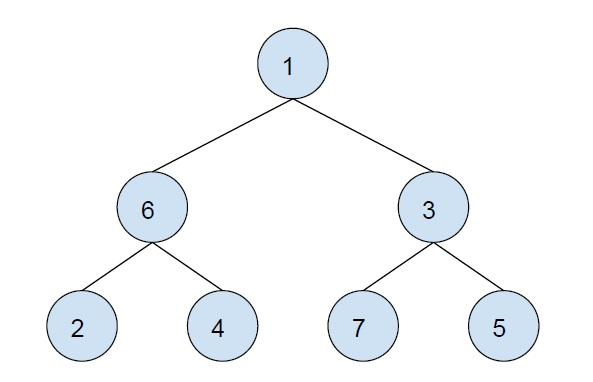# XOR of all the nodes in the sub-tree of the given node in C++

In this problem, we are given an n tree and there are some queries that are nodes of the tree. Our task is to print the XOR of all nodes of the sub-tree formed by the given node.

Let’s take an example to understand the problem,Queries − {1, 6, 5}

Output

0
0
5

Explanation

1^6^3^2^4^7^5
6^2^4
5

To solve this problem, we will compute the xor of all nodes of the sub-tree by traversing the tree once and store it. Now, we will compute the xor of all nodes of the sub-tree if the child nodes and then computing for all given sub-trees. Storing the results saves time.

## Example

Program to show the implementation of our solution,

Live Demo

#include <bits/stdc++.h>
using namespace std;
vector<vector<int> > graph;
vector<int> values, xorValues;
int computeXorValues(int i, int prev){
int x = values[i];
for (int j = 0; j < graph[i].size(); j++)
if (graph[i][j] != prev) {
x ^= computeXorValues(graph[i][j], i);
}
xorValues[i] = x;
return x;
}
int solveQuerry(int u){
return xorValues[u];
}
int main(){
int n = 7;
graph.resize(n);
xorValues.resize(n);
graph.push_back(1);
graph.push_back(2);
graph.push_back(3);
graph.push_back(4);
graph.push_back(5);
graph.push_back(6);
values.push_back(1);
values.push_back(2);
values.push_back(3);
values.push_back(4);
values.push_back(5);
values.push_back(6);
values.push_back(7);
computeXorValues(0, -1);
int queries[] = { 0, 2, 4, 6 };
int q = sizeof(queries) / sizeof(queries);
for (int i = 0; i < q; i++)
cout<<"Solution for querry "<<(i+1)<<": "<<solveQuerry(queries[i])<<endl;
return 0;
}

## Output

Solution for querry 1: 0
Solution for querry 2: 2
Solution for querry 3: 5
Solution for querry 4: 7